Levels »

# ...But They’re Hungry

### The Pyramid, Elevation: 9.0m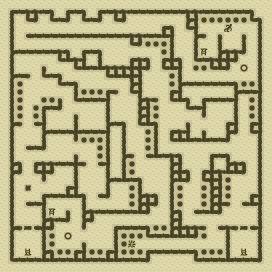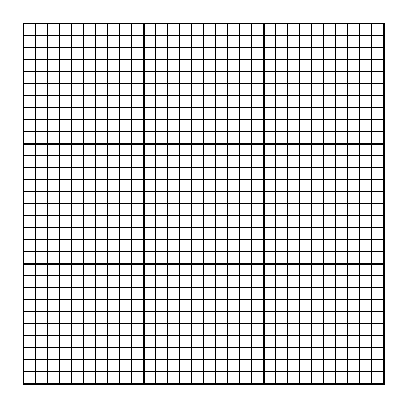### Items

•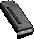10 Walther P4 Magazines (80 rounds) (27, 2) x8 (on Anonymous German) - (27, 2) x8 (on Anonymous German) - (27, 2) x8 (on Anonymous German) - (27, 2) x8 (on Anonymous German) - (27, 2) x8 (on Anonymous German) - (27, 13) x8 - (26, 15) x8 - (7, 21) x8 - (14, 22) x8 - (14, 22) x8

•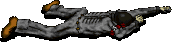(27, 2) Anonymous German
•Walther P4 Magazine x8
•Walther P4 Magazine x8
•Walther P4 Magazine x8
•Walther P4 Magazine x8
•Walther P4 Magazine x8

### Monsters

•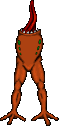15 Headless (12, 4) - (12, 5) - (1, 7) - (19, 9) - (23, 15) - (5, 16) - (6, 18) - (22, 18) - (18, 20) - (18, 21) - (13, 22) - (18, 22) - (3, 23) - (13, 23) - (12, 26)
•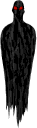2 Phantasms (1, 24) - (27, 25)
•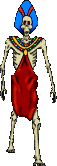42 Zombies (Random rate: 4) (1, 1) - (4, 1) - (8, 1) - (12, 1) - (13, 4) - (25, 4) - (28, 4) - (8, 5) - (13, 5) - (2, 7) - (12, 9) - (17, 9) - (18, 10) - (13, 12) - (25, 12) - (26, 12) - (6, 13) - (10, 13) - (29, 13) - (3, 15) - (1, 16) - (3, 16) - (6, 17) - (10, 17) - (29, 17) - (1, 18) - (22, 18) - (19, 19) - (9, 20) - (11, 20) - (2, 21) - (3, 22) - (17, 22) - (14, 23) - (22, 23) - (23, 23) - (28, 23) - (6, 24) - (11, 26) - (6, 28) - (9, 28) - (7, 29)

### Locations

• 2 Single-use (type 14) door closers (2, 26) - (29, 26)
• 2 Save runes(29, 7) - (7, 28)
• False wall(23, 29) east side